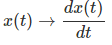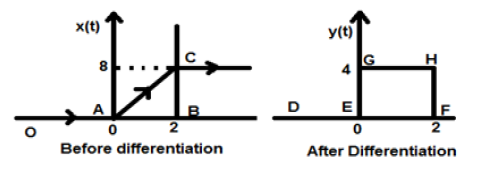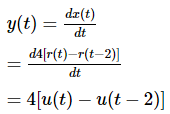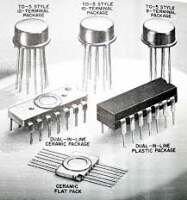Courses

Differentiation - Operations on Signals Notes | Study Digital Signal Processing - Electrical Engineering (EE)

Electrical Engineering (EE): Differentiation - Operations on Signals Notes | Study Digital Signal Processing - Electrical Engineering (EE)

The document Differentiation - Operations on Signals Notes | Study Digital Signal Processing - Electrical Engineering (EE) is a part of the Electrical Engineering (EE) Course Digital Signal Processing.
All you need of Electrical Engineering (EE) at this link: Electrical Engineering (EE)

Two very important operations performed on the signals are Differentiation and Integration.

Differentiation

Differentiation of any signal x(t) means slope representation of that signal with respect to time. Mathematically, it is represented as;In the case of OPAMP differentiation, this methodology is very helpful. We can easily differentiate a signal graphically rather than using the formula. However, the condition is that the signal must be either rectangular or triangular type, which happens in most cases.

Original SignalDifferentiated Signal
RampStep
StepImpulse
Impulse1

The above table illustrates the condition of the signal after being differentiated. For example, a ramp signal converts into a step signal after differentiation. Similarly, a unit step signal becomes an impulse signal.

Example

Let the signal given to us be x(t) = 4[r(t) − r(t − 2)]. When this signal is plotted, it will look like the one on the left side of the figure given below. Now, our aim is to differentiate the given signal.

To start with, we will start differentiating the given equation. We know that the ramp signal after differentiation gives unit step signal.So our resulting signal y(t) can be written as;Now this signal is plotted finally, which is shown in the right hand side of the above figure.

The document Differentiation - Operations on Signals Notes | Study Digital Signal Processing - Electrical Engineering (EE) is a part of the Electrical Engineering (EE) Course Digital Signal Processing.
All you need of Electrical Engineering (EE) at this link: Electrical Engineering (EE)Use Code STAYHOME200 and get INR 200 additional OFF Use Coupon Code

Top Courses for Electrical Engineering (EE)Digital Signal Processing

3 videos|50 docs|54 tests

Top Courses for Electrical Engineering (EE)Track your progress, build streaks, highlight & save important lessons and more!

,

,

,

,

,

,

,

,

,

,

,

,

,

,

,

,

,

,

,

,

,

;